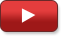Explore BrainMass

# An Introduction to Integration

This lecture will begin by defining the notion of anti-derivative and indefinite integral. Basic properties and the techniques of integration by parts and substitution will then be discussed. Examples, including the solution to simple differential equations that arise from motion in a constant gravitational field, will be given along the way. We will close with a discussion of area and the statement of the fundamental theorem of calculus. This lecture is ideal for first year calculus students who have learned the basics of differentiation.

In this series: 4 Videos | 00:33:07 Total Length

In Part 1 of the series, Academic Expert Mike Melko introduces the topic of Integration in Calculus, and discusses anti-derivatives and indefinite integrals.

Part #: 1 | 07:34The solution to two simple differential equations.

Part #: 2 | 09:25
\$2.49Techniques of Integration.

Part #: 3 | 08:37
\$2.49The relation between anti-derivatives and area.

Part #: 4 | 07:31
\$2.49